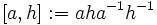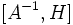# Subgroup normalizes its commutator with any subset

## Statement

### Statement with the right-action convention

Suppose$G$ is a group,$H$ is a subgroup of$G$, and$A$ is a subset of$G$. Then,$H$ normalizes the following subgroup of$G$:$[A,H] := \langle [a,h] \mid a \in A, h \in H \rangle$.

Here,$[a,h] := a^{-1}h^{-1}ah$ denotes the commutator of the two elements.

Note that since$[a,h]^{-1} = [h,a]$, the subgroup$[A,H]$ equals the subgroup$[H,A]$.

### Statement with the left-action convention

Suppose$G$ is a group,$H$ is a subgroup of$G$, and$A$ is a subset of$G$. Then,$H$ normalizes the following subgroup of$G$:$[A,H] := \langle [a,h] \mid a \in A, h \in H \rangle$.

Here,$[a,h] := aha^{-1}h^{-1}$ denotes the commutator of the two elements.

Note that since$[a,h]^{-1} = [h,a]$, the subgroup$[A,H]$ equals the subgroup$[H,A]$.

### Difference between the two statements

Note also that the subgroup$[A,H]$ defined in the right-action convention is the subgroup$[A^{-1},H]$ as per the left-action convention. However, the truth of the two statements is still equivalent since the quantification is over all subsets of$G$.

## Related facts

### Applications

• Product with commutator equals join with conjugate: If$H \le G$ and$g \in G$,$H[g,H] = \langle H, H^g \rangle$.
• Commutator of two subgroups is normal in join

## Facts used

1. Formula for commutator of element and product of two elements: With the right-action convention, this is expressed as:$\! [x,yz] = [x,z][x,y]^z$.

where$a^g = g^{-1}ag$. and$[a,b] = a^{-1}b^{-1}ab$.

With the left-action convention, it is expressed as:$\! [x,yz] = [x,y]c_y([x,z])$

where$[g,h] := ghg^{-1}h^{-1}$ and$c_g(h) := ghg^{-1}$.

## Proof

### With the right-action convention

Given: A group$G$, a subgroup$H$, a subset$A$ of$G$.

To prove:$H$ normalizes$[A,H]$.

Proof: Since$[a,h]$ (with$a \in A, h \in H$) form a generating set for$H$, it suffices to show that$[a,h]^k \in [A,H]$ for any$k \in H$. Let's do this. By fact (1), we have:$\! [a,hk] = [a,k][a,h]^k$.

This rearranges to give:$\! [a,h]^k = [a,k]^{-1}[a,hk]$.

Note that since$k,h \in H$,$hk \in H$. Thus, the right side is a left quotient of two elements in$[A,H]$, hence it is in$[A,H]$, completing the proof.